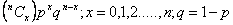# Statistics Assignment Help With Binomial Distribution

## 5.2 Binomial Distribution:

A random variable X is said to follow binomial distribution if it assumes only non-negative values and its probability mass function is given by

P(X=x) =p(x) =The two independent constants n and p in the distribution are known as the parameters of the distribution. ‘n’ is also sometimes, known as the degree of the binomial distribution.Binomial distribution is a discrete distribution as X can take only the integral values, viz., 0,1,2,…..,n. Any variable which follow binomial distribution is known as binomial variate.

We shall use the notation X~ B(n,p) to denote that the random variable X follows binomial distribution with parameters n and p.

The probability p(x) is also sometimes denoted by b(x,n,p).